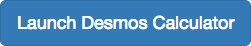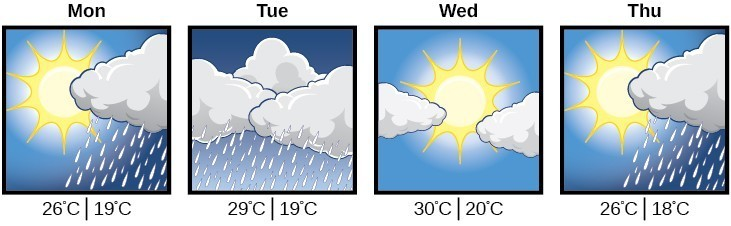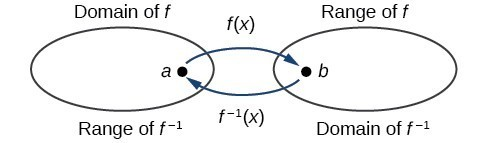## Characteristics of Inverse Functions

### Learning Outcomes

• Identify an inverse for tabular data.
• Test whether two functions are inverses.
• Determine the domain and range of an inverse.Suppose a fashion designer traveling to Milan for a fashion show wants to know what the temperature will be. He is not familiar with the Celsius scale. To get an idea of how temperature measurements are related, he asks his assistant, Betty, to convert 75 degrees Fahrenheit to degrees Celsius. She finds the formula $C=\frac{5}{9}\left(F - 32\right)$ and substitutes 75 for $F$ to calculate $\frac{5}{9}\left(75 - 32\right)\approx {24}^{ \circ} {C}$.Knowing that a comfortable 75 degrees Fahrenheit is about 24 degrees Celsius, he sends his assistant the week’s weather forecast for Milan, and asks her to convert all of the temperatures to degrees Fahrenheit.

At first, Betty considers using the formula she has already found to complete the conversions. After all, she knows her algebra, and can easily solve the equation for $F$ after substituting a value for $C$. For example, to convert 26 degrees Celsius, she could write

\begin{align}&26=\frac{5}{9}\left(F - 32\right) \\[1.5mm] &26\cdot \frac{9}{5}=F - 32 \\[1.5mm] &F=26\cdot \frac{9}{5}+32\approx 79 \end{align}

After considering this option for a moment, however, she realizes that solving the equation for each of the temperatures will be awfully tedious. She realizes that since evaluation is easier than solving, it would be much more convenient to have a different formula, one that takes the Celsius temperature and outputs the Fahrenheit temperature.

The formula for which Betty is searching corresponds to the idea of an inverse function, which is a function for which the input of the original function becomes the output of the inverse function and the output of the original function becomes the input of the inverse function.

Given a function $f\left(x\right)$, we represent its inverse as ${f}^{-1}\left(x\right)$, read as “$f$ inverse of $x$.” The raised $-1$ is part of the notation. It is not an exponent; it does not imply a power of $-1$ . In other words, ${f}^{-1}\left(x\right)$ does not mean $\frac{1}{f\left(x\right)}$ because $\frac{1}{f\left(x\right)}$ is the reciprocal of $f$ and not the inverse.

The “exponent-like” notation comes from an analogy between function composition and multiplication: just as ${a}^{-1}a=1$ (1 is the identity element for multiplication) for any nonzero number $a$, so ${f}^{-1}\circ f$ equals the identity function, that is,

$\left({f}^{-1}\circ f\right)\left(x\right)={f}^{-1}\left(f\left(x\right)\right)={f}^{-1}\left(y\right)=x$

This holds for all $x$ in the domain of $f$. Informally, this means that inverse functions “undo” each other. However, just as zero does not have a reciprocal, some functions do not have inverses.

Given a function $f\left(x\right)$, we can verify whether some other function $g\left(x\right)$ is the inverse of $f\left(x\right)$ by checking whether either $g\left(f\left(x\right)\right)=x$ or $f\left(g\left(x\right)\right)=x$ is true. We can test whichever equation is more convenient to work with because they are logically equivalent (that is, if one is true, then so is the other.)

For example, $y=4x$ and $y=\frac{1}{4}x$ are inverse functions.

$\left({f}^{-1}\circ f\right)\left(x\right)={f}^{-1}\left(4x\right)=\frac{1}{4}\left(4x\right)=x$

and

$\left({f}^{}\circ {f}^{-1}\right)\left(x\right)=f\left(\frac{1}{4}x\right)=4\left(\frac{1}{4}x\right)=x$

A few coordinate pairs from the graph of the function $y=4x$ are (−2, −8), (0, 0), and (2, 8). A few coordinate pairs from the graph of the function $y=\frac{1}{4}x$ are (−8, −2), (0, 0), and (8, 2). If we interchange the input and output of each coordinate pair of a function, the interchanged coordinate pairs would appear on the graph of the inverse function.

### A General Note: Inverse Function

For any one-to-one function $f\left(x\right)=y$, a function ${f}^{-1}\left(x\right)$ is an inverse function of $f$ if ${f}^{-1}\left(y\right)=x$. This can also be written as ${f}^{-1}\left(f\left(x\right)\right)=x$ for all $x$ in the domain of $f$. It also follows that $f\left({f}^{-1}\left(x\right)\right)=x$ for all $x$ in the domain of ${f}^{-1}$ if ${f}^{-1}$ is the inverse of $f$.

The notation ${f}^{-1}$ is read “$f$ inverse.” Like any other function, we can use any variable name as the input for ${f}^{-1}$, so we will often write ${f}^{-1}\left(x\right)$, which we read as $f$ inverse of $x$“.

Keep in mind that ${f}^{-1}\left(x\right)\ne \frac{1}{f\left(x\right)}$ and not all functions have inverses.

### Example: Identifying an Inverse Function for a Given Input-Output Pair

If for a particular one-to-one function $f\left(2\right)=4$ and $f\left(5\right)=12$, what are the corresponding input and output values for the inverse function?

### Try It

Given that ${h}^{-1}\left(6\right)=2$, what are the corresponding input and output values of the original function $h?$

### How To: Given two functions $f\left(x\right)$ and $g\left(x\right)$, test whether the functions are inverses of each other.

1. Determine whether $f\left(g\left(x\right)\right)=x$ and $g\left(f\left(x\right)\right)=x$.
2. If both statements are true, then $g={f}^{-1}$ and $f={g}^{-1}$. If either statement is false, then $g\ne {f}^{-1}$ and $f\ne {g}^{-1}$.

### Example: Testing Inverse Relationships Algebraically

If $f\left(x\right)=\dfrac{1}{x+2}$ and $g\left(x\right)=\dfrac{1}{x}-2$, is $g={f}^{-1}?$

### Try It

If $f\left(x\right)={x}^{3}-4$ and $g\left(x\right)=\sqrt{x+4}$, is $g={f}^{-1}?$

### Example: Determining Inverse Relationships for Power Functions

If $f\left(x\right)={x}^{3}$ (the cube function) and $g\left(x\right)=\frac{1}{3}x$, is $g={f}^{-1}?$

### Try It

If $f\left(x\right)={\left(x - 1\right)}^{3}\text{and}g\left(x\right)=\sqrt{x}+1$, is $g={f}^{-1}?$

## Determine the Domain and Range of an Inverse Function

The outputs of the function $f$ are the inputs to ${f}^{-1}$, so the range of $f$ is also the domain of ${f}^{-1}$. Likewise, because the inputs to $f$ are the outputs of ${f}^{-1}$, the domain of $f$ is the range of ${f}^{-1}$. We can visualize the situation.Domain and range of a function and its inverse

When a function has no inverse function, it is possible to create a new function where that new function on a limited domain does have an inverse function. For example, the inverse of $f\left(x\right)=\sqrt{x}$ is ${f}^{-1}\left(x\right)={x}^{2}$, because a square “undoes” a square root; but the square is only the inverse of the square root on the domain $\left[0,\infty \right)$, since that is the range of $f\left(x\right)=\sqrt{x}$.

We can look at this problem from the other side, starting with the square (toolkit quadratic) function $f\left(x\right)={x}^{2}$. If we want to construct an inverse to this function, we run into a problem, because for every given output of the quadratic function, there are two corresponding inputs (except when the input is 0). For example, the output 9 from the quadratic function corresponds to the inputs 3 and –3. But an output from a function is an input to its inverse; if this inverse input corresponds to more than one inverse output (input of the original function), then the “inverse” is not a function at all! To put it differently, the quadratic function is not a one-to-one function; it fails the horizontal line test, so it does not have an inverse function. In order for a function to have an inverse, it must be a one-to-one function.

In many cases, if a function is not one-to-one, we can still restrict the function to a part of its domain on which it is one-to-one. For example, we can make a restricted version of the square function $f\left(x\right)={x}^{2}$ with its range limited to $\left[0,\infty \right)$, which is a one-to-one function (it passes the horizontal line test) and which has an inverse (the square-root function).

If $f\left(x\right)={\left(x - 1\right)}^{2}$ on $\left[1,\infty \right)$, then the inverse function is ${f}^{-1}\left(x\right)=\sqrt{x}+1$.

• The domain of $f$ = range of ${f}^{-1}$ = $\left[1,\infty \right)$.
• The domain of ${f}^{-1}$ = range of $f$ = $\left[0,\infty \right)$.

Q & A

Is it possible for a function to have more than one inverse?

No. If two supposedly different functions, say, $g$ and $h$, both meet the definition of being inverses of another function $f$, then you can prove that $g=h$. We have just seen that some functions only have inverses if we restrict the domain of the original function. In these cases, there may be more than one way to restrict the domain, leading to different inverses. However, on any one domain, the original function still has only one unique inverse.

### A General Note: Domain and Range of Inverse Functions

The range of a function $f\left(x\right)$ is the domain of the inverse function ${f}^{-1}\left(x\right)$.

The domain of $f\left(x\right)$ is the range of ${f}^{-1}\left(x\right)$.

### How To: Given a function, find the domain and range of its inverse.

1. If the function is one-to-one, write the range of the original function as the domain of the inverse, and write the domain of the original function as the range of the inverse.
2. If the domain of the original function needs to be restricted to make it one-to-one, then this restricted domain becomes the range of the inverse function.

### Example: Finding the Inverses of Toolkit Functions

Identify which of the toolkit functions besides the quadratic function are not one-to-one, and find a restricted domain on which each function is one-to-one, if any. The toolkit functions are reviewed below. We restrict the domain in such a fashion that the function assumes all y-values exactly once.

 Constant Identity Quadratic Cubic Reciprocal $f\left(x\right)=c$ $f\left(x\right)=x$ $f\left(x\right)={x}^{2}$ $f\left(x\right)={x}^{3}$ $f\left(x\right)=\frac{1}{x}$ Reciprocal squared Cube root Square root Absolute value $f\left(x\right)=\frac{1}{{x}^{2}}$ $f\left(x\right)=\sqrt{x}$ $f\left(x\right)=\sqrt{x}$ $f\left(x\right)=|x|$

### Try It

The domain of the function $f$ is $\left(1,\infty \right)$ and the range of the function $f$ is $\left(\mathrm{-\infty },-2\right)$. Find the domain and range of the inverse function.

## Contribute!

Did you have an idea for improving this content? We’d love your input.